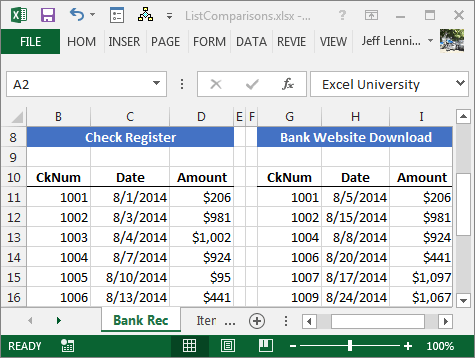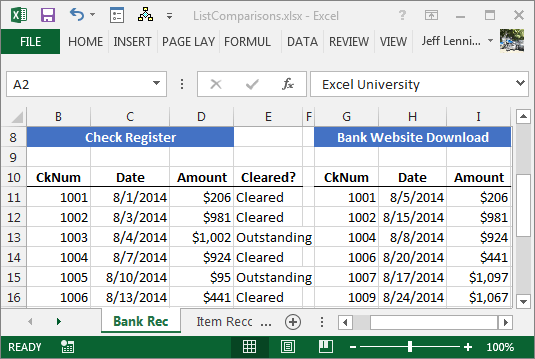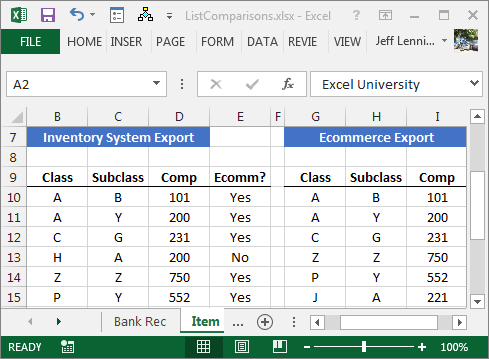# Comparing Spreadsheet Lists with Ease

Have you ever had two lists in Excel and wondered what items on one list appear on the other? For example, you’ve exported a check register from your accounting system and downloaded activity from your bank’s website, and you simply wanted to know which checks cleared the bank. That is, which checks on the checklist also appear on the bank download?

We’ll refer to this idea as list comparisons and, fortunately, Excel is a pro at them.

## List Comparisons

For our purposes, a list comparison is the task of comparing two Excel lists to find which items are the same or different between them. Consider the two lists in Figure 1.Figure 1

Our objective is to quickly determine which checks on the left side, the check register, also appear on the right side, the bank download. Since this is Excel, there are many options available. In this article, we’ll discuss my favorite functions for performing list comparisons: COUNTIFS and IF.

## COUNTIFS

This is a multiple-condition, counting function. Wait, what? It’s a counting function because it counts the number of cells in a range, but it only includes those rows that meet one or more conditions. The syntax for the function follows:

`=COUNTIFS(criteria_range1, criteria1,…)`

Where:

• Criteria_range1 is the range of cells to count.
• Criteria1 is the criteria the cell values must meet to be included in the count.
• means up to 127 conditions may be tested.

Let’s see how we can use this conditional counting function to help with a list comparison.
We can write a formula next to the check register list that counts how many times each check number appears in the bank download list. If the formula counts zero, then we determine the check is outstanding. If the formula returns one, then we determine the check has cleared the bank. The following formula written into cell E11 and filled down would provide such a result:

`=COUNTIFS(\$G\$11:\$G\$18,B11)`

Where:

• B11 is the check register check number we are seeking.

Now, let me ask you a question: What if, instead of returning the number of matching rows, we wanted to return something else, such as “Cleared” or “Outstanding.” This is where our friend IF can help.

## IF

The IF function returns a value based on the result of a test. The syntax follows:

`=IF(logical_test, [value_ if_true], [value_if_false])`

Where:

• logical_test is the expression to test.
• [value_if_true] is the value to return if logical_test is TRUE.
• [value_if_false] the value to return if logical_test is FALSE.

If we wanted the formula to return “Outstanding” if the count is zero, and “Cleared” otherwise, we could update the formula in E11 as follows:

`=IF(COUNTIFS(\$G\$11:\$G\$18,B11)=0,"Outstanding","Cleared")`

Where:

• COUNTIFS(\$G\$11:\$G\$18,B11)=0 is the logical test, does the count equal zero?
• “Outstanding” is the value to return if the count is equal to zero.
• “Cleared” is the value to return if otherwise.

After updating the formula and filling it down, the cleaner version of the bank reconciliation is shown in Figure 2.Figure 2

Now, let me ask you another question: What if we wanted to compare multiple columns instead of just using one? Good news, the COUNTIFS function supports multiple
conditions, and will return the total number of rows that meet all conditions.

Let’s say we have two lists of items: one from our inventory system and the other from our online ecommerce website. We need to compare them, but there isn’t a single unique product column. The unique product code is the combination of the class, subclass and component identification columns.

To compare them, we need to consider all three columns; fortunately, we can easily achieve this with COUNTIFS. For example, the following formula could be used in E10 and filled down:

`=IF(COUNTIFS(\$G\$10:\$G\$17,B10,\$H\$10:\$H\$17,C10,\$I\$10:\$I\$17,D10)=0,"No","Yes")`

Where:

• COUNTIFS(\$G\$10:\$G\$17,B10,\$H\$10:\$H\$17,C10,\$I\$10:\$I\$17,D10)=0 is the logical test, does the count equal zero?
• Where:
• \$G\$10:\$G\$17 is the first criteria range, the class column.
• B10 is the first criteria value, the class.
• \$H\$10:\$H\$17 is the second criteria range, the subclass column.
• C10 is the second criteria value, the subclass.
• \$I\$10:\$I\$17 is the third criteria range, the component column.
• D10 is the third criteria value, the component.
• “No” is the value to return if the count is zero.
• “Yes” is the value to return if the count is not zero.

The results are shown in Figure 3.Figure 3

That’s the basic idea of comparing lists based on one or more conditions. Excel can help us perform list comparisons quite easily. And remember, Excel rules!

ListComparisons.xlsx

Posted in### Jeff Lenning

I love sharing the things I've learned about Excel, and I built Excel University to help me do that. My motto is: Learn Excel. Work Faster.

### Excel is not what it used to be.

You need the Excel Proficiency Roadmap now. Includes 6 steps for a successful journey, 3 things to avoid, and weekly Excel tips.